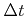Next: 4.3.2 Pruning and Consistency Up: 4.3 Sparse Field Implementation Previous: 4.3 Sparse Field Implementation

## 4.3.1 Time Integration

For the time integration step it is assumed that the defined grid points of the H-RLE data structure include at least all active grid points as well as all neighbors which are needed for the applied time integration scheme. Furthermore, at each active grid point the surface velocity field must be known. To update the LS values of all active grid points in time, first the time incrementneeds to be determined according to (3.33). This requires an iteration over the data structure using a finite difference stencil of iterators. At each active grid point the approximations to the Hamiltonian are calculated and stored in a separate array, because they are needed again, after the determination of the time increment, for updating the LS values.

After updating the LS values of all active grid points, the LS values of all other defined grid points are no longer needed. Hence, it is favorable to reduce the set of defined grid points. This can be performed during the same initial iteration. Every active grid point is inserted together with its LS value into a new H-RLE data structure. The signs of all non-active grid points are maintained and stored within the new H-RLE data structure as members of undefined runs. After the iteration, the memory used for storing the old H-RLE data structure can be freed.

Once the time increment is known and the approximations to the Hamiltonian are calculated for all active grid points, it is possible to update the LS values of all active grid points using (3.5). This operation could be realized by processing the data structure using a basic iterator. However, since the new H-RLE data structure only contains active grid points, the array holding the LS values has the same size as the array for the approximations to the Hamiltonian. Therefore, the update of the LS values can simply be implemented by a multiplication of the Hamiltonian array with the time incrementfollowed by a subtraction from the LS values array of the H-RLE data structure.Next: 4.3.2 Pruning and Consistency Up: 4.3 Sparse Field Implementation Previous: 4.3 Sparse Field Implementation

Otmar Ertl: Numerical Methods for Topography Simulation